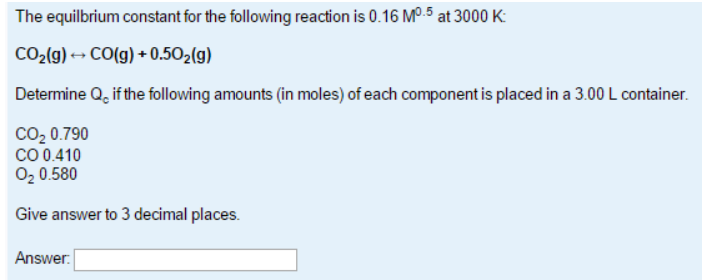# Problem: The equilibrium constant for the following reaction is 0.16 M 0.5 at 3000 K: CO2 (g) &lt;-&gt; CO (g) + 0.5O2(g) Determine Qc if the following amounts (in moles) of each component is placed in a 3.00 L container. CO2 0.790 CO 0.410 O2 0.580 Give answer to 3 decimal places.

###### FREE Expert Solution
89% (13 ratings)###### Problem Details

The equilibrium constant for the following reaction is 0.16 M 0.5 at 3000 K:

CO2 (g) <-> CO (g) + 0.5O2(g)

Determine Qc if the following amounts (in moles) of each component is placed in a 3.00 L container.

CO2 0.790

CO 0.410

O2 0.580

Give answer to 3 decimal places.